# The SURVEYLOGISTIC Procedure

### Displayed Output

Subsections:

The SURVEYLOGISTIC procedure produces output that is described in the following sections.

Output that is generated by the EFFECT, ESTIMATE, LSMEANS, LSMESTIMATE, and SLICE statements are not listed below. For information about the output that is generated by these statements, see the corresponding sections of Chapter 19: Shared Concepts and Topics.

#### Model Information

By default, PROC SURVEYLOGISTIC displays the following information in the "Model Information" table:

• name of the input Data Set

• name and label of the Response Variable if the single-trial syntax is used

• number of Response Levels

• name of the Events Variable if the events/trials syntax is used

• name of the Trials Variable if the events/trials syntax is used

• name of the Offset Variable if the OFFSET= option is specified

• name of the Frequency Variable if the FREQ statement is specified

• name(s) of the Stratum Variable(s) if the STRATA statement is specified

• total Number of Strata if the STRATA statement is specified

• name(s) of the Cluster Variable(s) if the CLUSTER statement is specified

• total Number of Clusters if the CLUSTER statement is specified

• name of the Weight Variable if the WEIGHT statement is specified

• Lower Bound DEFFBOUND parameter used in the VADJUST=MOREL(DEFFBOUND= ) option

• whether FPC (finite population correction) is used

#### Variance Estimation

By default, PROC SURVEYLOGISTIC displays the following variance estimation information in the "Variance Estimation" table:

• Method, which is the variance estimation method

• Lower Bound DEFFBOUND parameter specified in the VADJUST=MOREL(DEFFBOUND= ) option

• whether FPC (finite population correction) is used

• Number of Replicates, if you specify the VARMETHOD=BRR or VARMETHOD=JACKKNIFE option

• Number of Replicates Used, if you specify the VARMETHOD=BRR or VARMETHOD=JACKKNIFE option and some of the replicates are excluded due to unattained convergence

• Fay Coefficient, if you specify the VARMETHOD=BRR(FAY) method-option

• Replicate Weights input data set name, if you use a REPWEIGHTS statement

• whether Missing Levels are created for categorical variables by the MISSING option

• whether observations with Missing Values are included in the analysis by the NOMCAR option

#### Data Summary

By default, PROC SURVEYLOGISTIC displays the following information for the entire data set:

• Number of Observations read from the input data set

• Number of Observations used in the analysis

If there is a DOMAIN statement, PROC SURVEYLOGISTIC also displays the following:

• Number of Observations in the current domain

• Number of Observations not in the current domain

If there is a FREQ statement, PROC SURVEYLOGISTIC also displays the following:

• Sum of Frequencies of all the observations read from the input data set

• Sum of Frequencies of all the observations used in the analysis

If there is a WEIGHT statement, PROC SURVEYLOGISTIC also displays the following:

• Sum of Weights of all the observations read from the input data set

• Sum of Weights of all the observations used in the analysis

• Sum of Weights of all the observations in the current domain, if DOMAIN statement is also specified.

#### Response Profile

By default, PROC SURVEYLOGISTIC displays a "Response Profile" table, which gives, for each response level, the ordered value (an integer between one and the number of response levels, inclusive); the value of the response variable if the single-trial syntax is used or the values "EVENT" and "NO EVENT" if the events/trials syntax is used; the count or frequency; and the sum of weights if the WEIGHT statement is specified.

#### Class Level Information

If you use a CLASS statement to name classification variables, PROC SURVEYLOGISTIC displays a "Class Level Information" table. This table contains the following information for each classification variable:

• Class, which lists each CLASS variable name

• Value, which lists the values of the classification variable. The values are separated by a white space character; therefore, to avoid confusion, you should not include a white space character within a classification variable value.

• Design Variables, which lists the parameterization used for the classification variables

#### Stratum Information

When you specify the LIST option in the STRATA statement, PROC SURVEYLOGISTIC displays a "Stratum Information" table, which provides the following information for each stratum:

• Stratum Index, which is a sequential stratum identification number

• STRATA variable(s), which lists the levels of STRATA variables for the stratum

• Population Total, if you specify the TOTAL= option

• Sampling Rate, if you specify the TOTAL= or RATE= option. If you specify the TOTAL= option, the sampling rate is based on the number of nonmissing observations in the stratum.

• N Obs, which is the number of observations

• number of Clusters, if you specify a CLUSTER statement

#### Maximum Likelihood Iteration History

The "Maximum Likelihood Iterative Phase" table gives the iteration number, the step size (in the scale of 1.0, 0.5, 0.25, and so on) or the ridge value, –2 log likelihood, and parameter estimates for each iteration. Also displayed are the last evaluation of the gradient vector and the last change in the –2 log likelihood. You need to use the ITPRINT option in the MODEL statement to obtain this table.

#### Score Test for the Parallel Lines Assumption

The "Score Test" table displays the score test result for testing the parallel lines assumption, if an ordinal response model is fitted. If LINK=CLOGLOG or LINK=PROBIT, this test is labeled "Score Test for the Parallel Slopes Assumption." The proportion odds assumption is a special case of the parallel lines assumption when LINK=LOGIT. In this case, the test is labeled "Score Test for the Proportional Odds Assumption." See the section Testing the Parallel Lines Assumption for more information.

#### Model Fit Statistics

By default, PROC SURVEYLOGISTIC displays the following information in the "Model Fit Statistics" table:

• "Model Fit Statistics" and "Testing Global Null Hypothesis: BETA=0" tables, which give the various criteria (–2 Log L, AIC, SC) based on the likelihood for fitting a model with intercepts only and for fitting a model with intercepts and explanatory variables. If you specify the NOINT option, these statistics are calculated without considering the intercept parameters. The third column of the table gives the chi-square statistics and p-values for the –2 Log L statistic and for the Score statistic. These test the joint effect of the explanatory variables included in the model. The Score criterion is always missing for the models identified by the first two columns of the table. Note also that the first two rows of the Chi-Square column are always missing, since tests cannot be performed for AIC and SC.

• generalized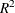measures for the fitted model if you specify the RSQUARE option in the MODEL statement

#### Type III Analysis of Effects

PROC SURVEYLOGISTIC displays the "Type III Analysis of Effects" table if the model contains an effect involving a CLASS variable. This table gives the degrees of freedom, the Wald Chi-square statistic, and the p-value for each effect in the model.

#### Analysis of Maximum Likelihood Estimates

By default, PROC SURVEYLOGISTIC displays the following information in the "Analysis of Maximum Likelihood Estimates" table:

• maximum likelihood estimate of the parameter

• estimated standard error of the parameter estimate, computed as the square root of the corresponding diagonal element of the estimated covariance matrix

• t value, which is the t statistic for testing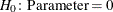• Pr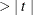, which is the two-sided p-value for the t test

•% confidence intervals for estimated parameters. You need to specify the CLPARM option in the MODEL statement to display these estimates.

• standardized estimate for the slope parameter, given by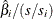, where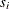is the total sample standard deviation for the ith explanatory variable and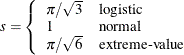You need to specify the STB option in the MODEL statement to obtain these estimates. Standardized estimates of the intercept parameters are set to missing.

• value of (e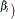for each slope parameter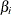if you specify the EXPB option in the MODEL statement. For continuous variables, this is equivalent to the estimated odds ratio for a one-unit change.

• label of the variable (if space permits) if you specify the PARMLABEL option in the MODEL statement. Because of constraints on the line size, the variable label might be suppressed in order to display the table in one panel. Use the SAS system option LINESIZE= to specify a larger line size to accommodate variable labels. A shorter line size can break the table into two panels, allowing labels to be displayed.

#### Odds Ratio Estimates

The "Odds Ratio Estimates" table displays the odds ratio estimates and the corresponding 95% Wald confidence intervals. For continuous explanatory variables, these odds ratios correspond to a unit increase in the risk factors.

#### Association of Predicted Probabilities and Observed Responses

The "Association of Predicted Probabilities and Observed Responses" table displays measures of association between predicted probabilities and observed responses, which include a breakdown of the number of pairs with different responses, and four rank correlation indexes: Somers’ D, Goodman-Kruskal Gamma, and Kendall’s Tau-a, and c.

#### Estimated Covariance Matrix

PROC SURVEYLOGISTIC displays the following information in the "Estimated Covariance Matrix" table:

• estimated covariance matrix of the parameter estimates if you use the COVB option in the MODEL statement

• estimated correlation matrix of the parameter estimates if you use the CORRB option in the MODEL statement

#### Linear Hypotheses Testing Results

The "Linear Hypothesis Testing" table gives the result of the Wald test for each TEST statement (if specified).